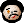# 2010-11-23 Changing the layout of theorems in beamer (en)

Continuing my previous post, let’s answer another of my friend’s questions on beamer. This time, he’d like to change the layout of theorem headings. (Notice that theorem bodies are easier to customize, since beamer uses amsthm and its concept of theorem styles internally—see documentation of amsthm for information on how to use or define theorem styles.)

It turns out that beamer has a nice hook enabling users defining their own layout of theorem headings. Let us look into a simple example:

\documentclass{beamer}

\makeatletter
\setbeamertemplate{theorem begin}
{%
\begin{\inserttheoremblockenv}
{%
\inserttheoremname
\inserttheoremnumber
\else
\fi
\inserttheorempunctuation
}%
}
\setbeamertemplate{theorem end}{\end{\inserttheoremblockenv}}
\makeatother

\begin{document}

\begin{frame}
\begin{theorem}[An example of beamer tricks]
This is a theorem.
\end{theorem}
\end{frame}

\end{document}


Notice how \makeatletter is needed here for the internal LaTeX command \@empty to work. Also notice that Computer Modern Sans Serif fonts do not contain bold italic (!), so \textmd is also necessary. Finally, notice the percentage signs effectively cancelling the newlines, which otherwise might get converted into spaces; in this case they most probably won’t be needed (well, to be sure one would have to read the beamer source), but they should never hurt (well, except some really strange cases; for instance, each numerical constant given to TeX’s low-level commands should be terminated by a space).

So, happy TeXing (or beamering?)!